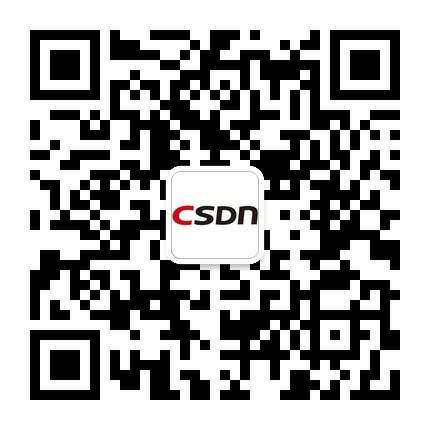# 12个有趣的C语言面试题

#### 发表于2012-09-06 09:16| 次阅读| 来源CSDN| 0 条评论| 作者王然

1.gets()函数

`#include<stdio.h> int main(void) {     char buff;     memset(buff,0,sizeof(buff));      gets(buff);      printf("\n The buffer entered is [%s]\n",buff);      return 0; } `

2.strcpy()函数

`#include<stdio.h>  int main(int argc, char *argv[]) {     int flag = 0;     char passwd;      memset(passwd,0,sizeof(passwd));      strcpy(passwd, argv);      if(0 == strcmp("LinuxGeek", passwd))     {         flag = 1;     }      if(flag)     {         printf("\n Password cracked \n");     }     else     {         printf("\n Incorrect passwd \n");      }     return 0; } `

`\$ ./psswd aaaaaaaaaaaaa  Password cracked `

3.main()的返回类型

`#include<stdio.h>  void main(void) {     char *ptr = (char*)malloc(10);      if(NULL == ptr)     {         printf("\n Malloc failed \n");         return;     }     else     {         // Do some processing         free(ptr);     }      return; } `

4.内存泄露

`#include<stdio.h>  void main(void) {     char *ptr = (char*)malloc(10);      if(NULL == ptr)     {         printf("\n Malloc failed \n");         return;     }     else     {         // Do some processing     }      return; } `

5.free()函数

`#include<stdio.h>  int main(int argc, char *argv[]) {     char *ptr = (char*)malloc(10);      if(NULL == ptr)     {         printf("\n Malloc failed \n");         return -1;     }     else if(argc == 1)     {         printf("\n Usage  \n");     }     else     {         memset(ptr, 0, 10);          strncpy(ptr, argv, 9);          while(*ptr != 'z')         {             if(*ptr == '')                 break;             else                 ptr++;         }          if(*ptr == 'z')         {             printf("\n String contains 'z'\n");             // Do some more processing         }         free(ptr);     }      return 0; } `

6.使用_exit退出

`#include<stdio.h>  void func(void) {     printf("\n Cleanup function called \n");     return; }  int main(void) {     int i = 0;      atexit(func);      for(;i<0xffffff;i++);      _exit(0); } `

### 本文导读

12个有趣的C语言面试题
• CSDN官方微信
• 扫描二维码,向CSDN吐槽
• 微信号：CSDNnews程序员移动端订阅下载